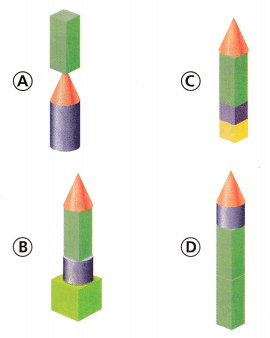# Texas Go Math Grade 3 Lesson 15.5 Answer Key Three-Dimensional Solids

Refer to our Texas Go Math Grade 3 Answer Key Pdf to score good marks in the exams. Test yourself by practicing the problems from Texas Go Math Grade 3 Lesson 15.5 Answer Key Three-Dimensional Solids.

## Texas Go Math Grade 3 Lesson 15.5 Answer Key Three-Dimensional Solids

Unlock the Problem

Solid figure have length, width, and height. They are also called three-dimensional figures.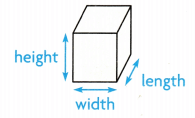Find solid figures.

Carly has a wooden clown puppet. Which parts of the puppet are shaped like a rectangular prism?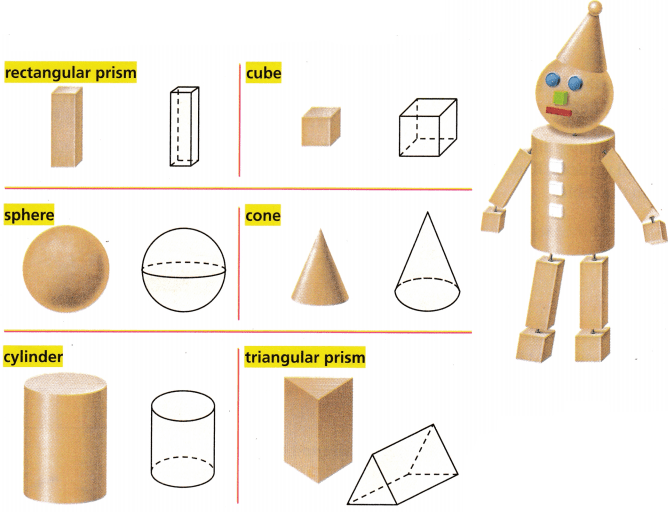So, the __________ are shaped like a rectangular prism.
So, the legs  are shaped like a rectangular prism.

Math Talk
Mathematical Processes

Give an example of an object in the classroom that has both curved surfaces.

Question 1.
What part of the puppet is shaped like a triangular prism?
Explanation:
The hands of the puppet is shaped like a triangular prism

Explore solid figures.

Solid figures can be classified by the number of faces, edges, and vertices.

A polygon that is a flat surface of a solid figure is a face.

An edge is a line segment formed where two faces meet.

A vertex is a point where three or more edges meet. The plural of vertex is vertices.

Try This! Write the word to describe what the arrow is pointing to.Explanation:
Written the words to describe the arrow is pointing to.Activity

Name the faces of prisms

Materials; cube, rectangular prism, triangular prism, paper, crayons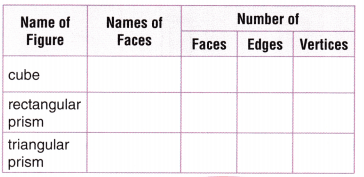• Trace the faces of a cube. Name the plane figures you drew.
• Count the number of faces, edges, and vertices. Record the numbers in the table.
• Repeat the steps for a rectangular prism and a triangular prism.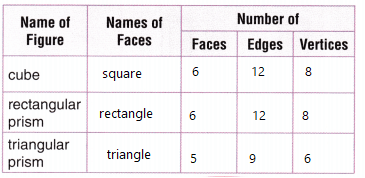Explanation:
Written the Table with the details

Math Talk
Mathematical Processes

Explain why a cube has the same number of faces, edges, and vertices as a rectangular prism.
As they are Identical in number of faces and edges and vertices,
The number of sides are equal

Share and Show

Question 1.
Name the solid figure that has the faces shown at the right.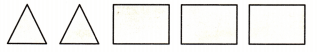Triangular prism
Explanation:
The faces of triangular prism has 2 triangle faces and 3 rectangular faces

Name the solid figure that the object is shaped like.

Question 2.Explanation:
A cylinder has 3 faces, 2 edges and 0 vertices

Question 3.Explanation:
Rectangular prism has 6 faces, 12 edges and 8 vertices

Question 4.Explanation:
Cone has 2 faces, 1 edges and 1 vertex

Question 5.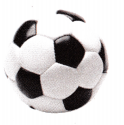A sphere has 1 face and 1 edge and 0 vertex

Name the solid figure. Then write the number of faces, edges, and vertices.

Question 6.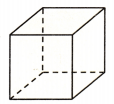__________ faces
__________ edges
__________ vertices
6 faces,
12 edges and
8 vertices
Explanation:
A symmetrical three-dimensional shape, either solid or hollow, contained by six equal squares.

Question 7.
Write one way you can sort a sphere, a cone, and a cylinder.
Explanation:
They all are prisms sorted in one word

H.O.T. Write All, Some, or None to complete the sentence.

Question 8.
___________ of the faces of a cube are squares.
Explanation:
6 of the faces of a cube are squares.

Question 9.
___________ of the faces of a triangular prism are triangles.
Explanation:
2 of the faces of a triangular prism are triangles.

Question 10.
___________ of the faces of a rectangular prism is curved.
Explanation:
There will not be any curved faces in a rectangular prism

Unlock the Problem

Question 11.
H.O.T. Multi-Step Analyze Fiona drew the Venn diagram at the right to help her sort solid figures. Where would the figure below be placed in the Venn diagram?a. What do you need to find?
Answer: In solid figures with curved surfaces the cone is drawn

b. What information are you given?
Answer: About Solid figures with flat surfaces and solid figures with curved surfaces

c. How will you solve the problem?
Answer: The cone has a curved surface

d. Complete the sentences.
I know that a cone has a _________ surface and a surface.
So, a cone goes ___________
I know that a cone has a curved surface and a surface.
So, a cone goes solid figures with curved surface

For 12 and 14, fill in the bubble completely to show your answer.

Question 12.
Multi-Step Scarlet and Violet take turns stacking blocks. They start with a cube. Next, Scarlet adds a cylinder. Violet puts a rectangular prism on top. Scarlet takes off the rectangular prism. She adds another cube. What does the structure look like?Explanation:
Scarlet and Violet take turns stacking blocks.
Next, Scarlet adds a cylinder. Violet puts a rectangular prism on top.
Scarlet takes off the rectangular prism. She adds another cube.
It looks like option A

Question 13.
Representations Joseph wants to sort the solid figures below into five groups. Use what you know about solid figures to complete the table.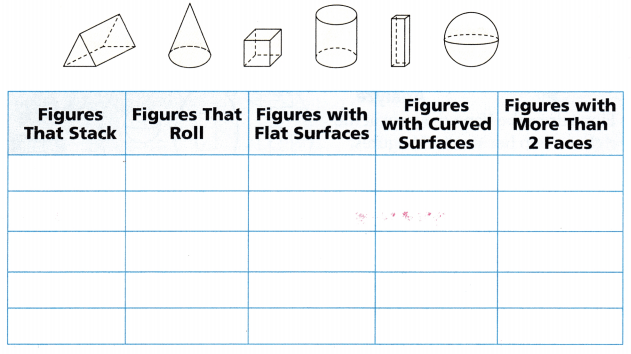Explanation:
Joseph  sorted the solid figures below into five groups.

Texas Test Prep

Question 14.
Aaron drew the figure at the right. What solid figure did he draw?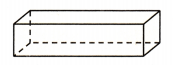(A) cube
(B) cone
(C) rectangular prism
(D) cylinder
Explanation:
Aaron drawn a rectangular prism

### Texas Go Math Grade 3 Lesson 15.5 Homework and Practice Answer Key

Name the solid figure that the object is shaped like.

Question 1.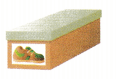Explanation:
Rectangular prism has 6 faces, 12 edges and 8 vertices

Question 2.Explanation:
A cylinder has 3 faces, 2 edges and 0 vertices

Question 3.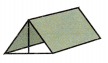Explanation:
Triangular prism has 5 faces, 9 edges and 6 vertices

Name the solid figure. Then write the number of faces, edges, and vertices.

Question 4.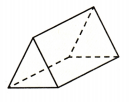__________ faces
__________ edges
__________ vertices
5 faces,
9 edges and
6 vertices
Explanation:

• Number of faces = 5
• Number of edges = 9
• Number of vertices = 6
• Shape of the base = Triangular
• Shape of Sides = Rectangular
• Surface Area = 2(Area of triangular bases)+Perimeter of base x Height of prism
• Volume = Area of base x Height of Prism

Question 5.__________ faces
__________ edges
__________ vertices
6 faces,
12 edges and
8 vertices
Explanation:

• A rectangular prism has 8 vertices, 12 sides and 6 rectangular faces.
• All the opposite faces of a rectangular prism are equal.
• A rectangular prism has a rectangular cross section.

Rectangular prisms can be of two types, namely right rectangular prism and non-right rectangular prisms.

Problem Solving

Question 6.
Amber drew this Venn diagram. Can Amber place a cone or a cylinder in the diagram? Explain.Explanation:
The number of faces and number of vertices  does not match to
the number of faces and vertices of a cone and cylinder

Question 7.
Mathias says that all of the faces of a triangular prism are triangles. Is his statement correct? Explain.
Explanation:
Only 2 faces are triangles and the other are rectangles

Lesson Check

Question 8.
Pia is making a rectangular prism from cutouts. She has these figures.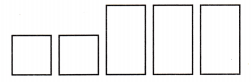Which flat figure does Pia need to complete the solid figure?
(A) square
(B) rectangle
(C) triangle
(D) circle
Explanation:
For making a rectangular prism we need 2 squares and 4 rectangles

Question 9.
Trent is making a triangular prism from cutouts. He has 2 triangles and 2 rectangles. Which flat figure does Trent need to complete the solid figure?Explanation:
A triangular prism has 1 triangle face and 3 rectangular faces
so, we need a rectangle.

Question 10.
Multi-Step Victor stacks some solid figures. He starts with a cylinder. Then he puts a cube on top. He places a triangular prism on top of the cube. Then he takes away the prism and adds a cone. What does Victor’s structure look like?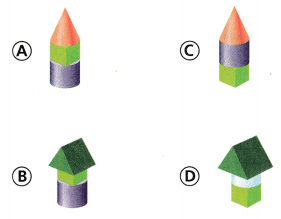Explanation:
Victor stacks some solid figures.
He starts with a cylinder.
Then he puts a cube on top.
He places a triangular prism on top of the cube.
he takes away the prism and adds a cone.
It looks like option A

Question 11.
Multi-Step Yoshi stacks these figures from bottom to top: cube, cylinder, rectangular prism, cone. He removes the cylinder and replaces it with a cube. What does Yoshi’s structure look like?